start page
This website is constantly being improved.
Old version

# Electromagnetic nature of gravity

### The variables are only explained for gravity below.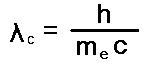The number of all nucleons N in a body is calculated by the following simple formula: N = m / u m - the mass of a body u - the atomic mass unit F - Gravity in [ N ] (Newton) r - Distance between the centers of bodies in [m] Im - Energy density of electromagnetic radiation from black bodies at a temperature of 3°K in [ J / m3 ] Im = 4,2 10 -14 [J / m3]) N1 - is the total number of nucleons in the first body N2 - is the total number of nucleons in the second body I used the following formulas to calculate energy density I: I = (P/A)/c P/A = a T4 Where: P - Radiation power of one body A - Area in which the radiation is emitted c - Speed of light in a vacuum a - Steffen-Bolzmann is constant a = 5,67 10-8 W/(m2K4)

It can be assumed that the energy density of microwave radiation is the same as the value of Im in the whole universe, because he has something in common with the background radiation. I suspect that the background radiation is responsible for all interaction types. My electromagnetic theory of gravity is easier to expand to a unified theory of all the fundamental forces of physics than previous theories. I have called it the "electromagnetic theory of gravity" because the value of 0.0186 x (Compton wavelength) high two ( 0,0186 λc2) - an average value of scattering cross section for inelastic scattering of microwaves could be on a nucleon in a certain solid angle range. The size "Im" represents the density of electromagnetic waves. The size "Im" represents the density of electromagnetic waves. All this leaves no doubt that gravity is a result of an interaction of electromagnetic waves and matter, if the mathematical formula had correctly reproduced the reality of the type of force. I wrote the values of some physical constants under "Constants", I used here for calculations.
15.05.2018
I derived formula # 1 from the following general formulas about which I stumbled in the theory of Mr. Steffen Kühn under the following link: Quantino theory. I suppose that is a source of electromagnetic radiation, such as. the sun, or any mass, only by the number of photons an influence on all surrounding bodies takes. Thus, the force acting on the bodies is not directly dependent on the source of the radiation, when we deal with very many bodies and / or very distant bodies. Then one could represent the force following: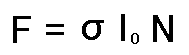Formula No. 0.

I can look at remote forces slightly differently (under (0)) than the forces for small distances between two bodies or particles, as below, where I had entered the number of interacting particles in both bodies N1 und N2.

### F = σ I N1 N2         (0a)

To test this formula, I calculated the unknown cross section for any two masses M and m by taking the force of formula (0a). compared with Newton's formula for gravitation between two bodies, as shown below: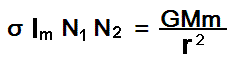Equation No. 1

From this I could calculate the following: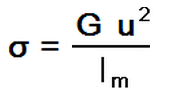G is the gravitational constant here.
In most cases one can represent the cross-section with a square of a certain fixed length, e.g. from λc oder re, where we talk about the length of the Compton wave of an electron or the "radius" of the electron that can be calculated with the following formula.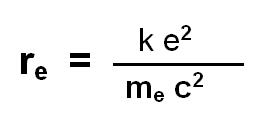Formula No. 2
and:Formula No. 3

k - Coulomb constant in [V*m /A*s]
ε0 - electric field constant in [ F / m]
e - elementary charge (charge of the electron) in [C]
me - mass of the electron in [kg] re - electromagnetic radius of the electron in [m]
A cross section is thus a multiplication of one of the quantities λcc oder re squared with a value of a function of the wave frequency ω, that is,f(ω)
This can be represented as follows:

oder

### σ = f(ω) (re)2         (5)

The cross section is mostly dependent on frequency, ie energy of particles acting on each other. But he has to have an average size when it comes to many particles, the forces would be transmitted or the forces are measured at longer intervals (according to the interaction). This also has & sigma; a certain average value for a given interaction. After some simplifications, I had the following formula:Der Wirkungsquerschnitt Sigma (σ) ist gleich für alle Körper im Universum und beträgt: The cross section sigma (σ) is the same for all bodies in the universe and is:
σ = 1,2 10-50 m2
I can represent the value either as a multiplication by the formula (4) or (5).
I had decided on the presentation under formula (4)
With the value of & sigma; I am very close to the fourth power of the Compton wavelength of an electron, which is:
λc4 = (2,4263102367 10-12 m)4 = 3,4656471 10-47 m4
and then it is
σ = (0,01876)2 λc4 = (0,01876 λc2)2
f(ω)= 0,01876
According to the definition (4), I must have the & sigma; in square, because the λc occurs in power four. So I can calculate the function f(ω), as in formula 6 below: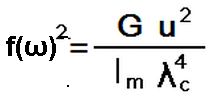Formula No. 6

For electric forces I had the following formula with the same method: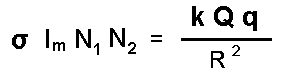Equation 2

Where:
N1 = Q / e
N2 = q / e
Here e is the value of elementary charge.
This equation can also be reduced and from this comes a new Formula 7 for Coulob force, as below with an adjustment factor A equal to 1 m2: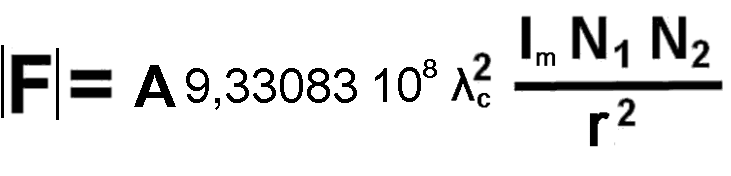Formula 7

For electrostatic interaction, the function f(ω) has the average value of
f(ω) = 2,55 109
So you can similarly represent the two interactions and a single radiation as the cause of the two forces. That would be a microwave radiation whose temperature almost equals the temperature of background radiation.
Are the two formulas a representation of reality or just a coincidence?
I would dare an experiment if the energy density of microwave radiation is actually an influence on the electric force between two charged bodies.